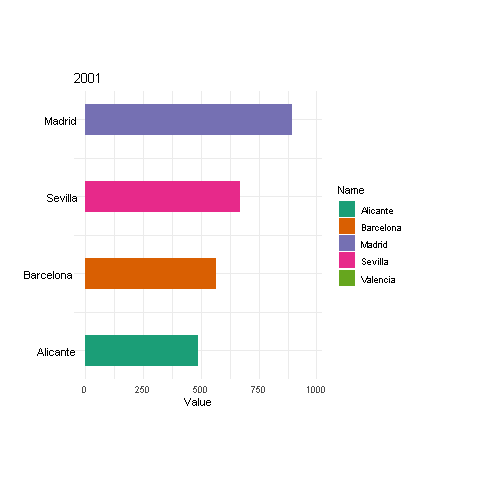# Reordering bars in GGanimate visualization

Dynamic ggplots with GGanimate! In this post we code evolution of a barplot over time and reordering its bar. ¡Click on and discover it!Last week several gganimate visualizations came to my feed. Some R users were wondering about reordering gganimate and ggplot2 bars as long as them are evolving (over animation time).

Then, we came up with this R viz where several bars are not only evolving and reordering over time but leaving and joining the chart. We want the top 4 countries over time, then the remaining countries in each timestamp should leave.

You can achieve this effect and this kind of gganimate visualization following this commented code:

``````library(ggplot2)
library(gganimate)
library(tidyverse)
"Valencia","Alicante",
"Sevilla"),5),
Year = factor(sort(rep(2001:2005, 5))),
Value = runif(25,100,1000))

df_evolution_data_filtered <- df_evolution_data %>%
group_by(Year) %>%
mutate(Rank = rank(Value)) %>% # Rank 1 the lowest 5 the higest
filter(Rank >= 2)              # Top 4 countries``````
``````ggplot(df_evolution_data_filtered) +
geom_col(aes(x=Rank, y=Value,
group=Name, fill=Name),
width=0.4) +
geom_text(aes(x=Rank, y=0,
label=Name, group=Name),
hjust=1.25) +
theme_minimal() + ylab('Value') +
theme(axis.title.y = element_blank(),
axis.text.y = element_blank(),
axis.ticks.y = element_blank(),
plot.margin = unit(c(5,5,5,5),
'lines')) +
scale_fill_brewer(palette="Dark2") +
coord_flip(clip='off') +
ggtitle('{closest_state}') +             # title with the timestamp period
transition_states(Year,
transition_length = 1,
state_length = 1) +
exit_fly(x_loc = 0, y_loc = 0) +         # chart exit animation params
enter_fly(x_loc = 0, y_loc = 0)          # chart enter animation params``````

Tune up your R visualizations on TypeThePipe